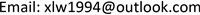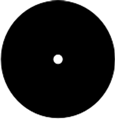﻿ 红外成像系统光学性能评估综述 Review of Optical Performance Testing of Thermal Infrared Imaging System

Optoelectronics
Vol.07 No.04(2017), Article ID:23008,10 pages
10.12677/OE.2017.74017

Review of Optical Performance Testing of Thermal Infrared Imaging System

Liwei Xu1, Qiang Li1, Huan Zhang2

1Photoelectric Specialty, Department of Control Engineering, Academy of Armored Force, Beijing

2Fire Control Specialty, Department of Control Engineering, Academy of Armored Force, BeijingReceived: Nov. 22nd, 2017; accepted: Dec. 6th, 2017; published: Dec. 13th, 2017ABSTRACT

In recent years, the evaluation of infrared imaging system is always a hot topic. It is an essential part of the development of infrared imaging system. The characteristics of infrared imaging system are analyzed, and the status of infrared camera system classification is introduced. With the development of time, the article introduces the development of infrared imaging system evaluation. Signal transfer function, the minimum resolution temperature difference of the system, modulation transfer function of the system and noise equivalent temperature difference are summarized and researched. Summed up the test methods are used frequently.

Keywords:Thermal Infrared Imaging System, Performance Evaluation, SiTF, MRTD, MTF, NETD

1陆军装甲兵学院控制工程系光电工程教研室，北京

2陆军装甲兵学院控制工程系火控工程教研室，北京Copyright © 2017 by authors and Hans Publishers Inc.1. 引言

2. 红外成像系统类型和基本组成

3. 红外成像系统性能评估方法及其发展

NVTherm性能模型是在1999年形成，该模型优于FLIR92模型，能够更好的对MRTD参数进行评估，对于目标的识别特性有着更加精确的评估。

TOD测试方法与Johnson准则相类似，其中大气对于红外辐射的作用影响被考虑在内，场景中目标所拥有的各种特性，还有整个系统中所有固有的各种性能参数、成像因素，研究人员通过利用三角形的阈值可分辨温差来将以上两方面因素联系起来，不同的探测概率、作用距离远近的分辨等级也就由此测量评估。

4. 红外成像系统检测主要参数研究

4.1. 信号传递函数SiTF

$\Delta {V}_{SYS}\text{=}\left[G\underset{{\lambda }_{1}}{\overset{{\lambda }_{2}}{\int }}R\left(\lambda \right)\frac{{A}_{\text{d}}}{4{F}^{2}}\frac{\partial {M}_{\text{e}}}{\partial T}{T}_{SYS}\left(\lambda \right){T}_{TEST}\text{d}\lambda \right]\Delta T$ (1)

$SiTF=\frac{\Delta {V}_{SYS}}{\Delta T}$ (2)Figure 1. Figure of Square hole targetFigure 2. Figure of Round hole target

$\Delta {V}_{SYS}=SiTF\Delta T+{V}_{\text{offset}}$ (3)

${V}_{\text{offset}}=\stackrel{¯}{\Delta V}-SiTF\stackrel{¯}{\Delta T}$ (4)

$\stackrel{¯}{\Delta T}=\frac{\underset{i=1}{\overset{N}{\sum }}\Delta {T}_{i}}{N}$ (5)

$\stackrel{¯}{\Delta V}=\frac{\underset{i=1}{\overset{N}{\sum }}\Delta {V}_{i}}{N}$ (6)

$SiTF=\frac{N\underset{i=1}{\overset{N}{\sum }}\Delta {V}_{i}\Delta {T}_{i}-\underset{i=1}{\overset{N}{\sum }}\Delta {V}_{i}\underset{i=1}{\overset{N}{\sum }}\Delta {T}_{i}}{N\underset{i=1}{\overset{N}{\sum }}{\left(\Delta {T}_{i}\right)}^{2}-{\left(N\underset{i=1}{\overset{N}{\sum }}\Delta {T}_{i}\right)}^{2}}$ (7)

4.2. 噪声等效温差NETD

$NETD=\frac{\Delta T}{S/\sigma }$ (8)

$NETD=\frac{\sigma }{SiTF}$ (9)

4.3. 调制传递函数MTF

$PS{D}_{in}\left(\epsilon ,\eta \right)={|MTF|}^{2}\cdot PS{D}_{out}\left(\epsilon ,\eta \right)$ (10)

4.4. 最小可分辨温差MRTD

MRTD一直以来的预测和测量就存在着矛盾，矛盾的原因在于预测模型并没有考虑到测量时的探测器增益变化，但是增益和对比度的调整使得测量值发生了变化，导致预测值与测量值的不相符，那么只有在预测时考虑到这些，针对MRTD模型中的缺陷进行完善，以求的更为切合实测数据的模型。

MRTD测试技术分为主观MRTD测试和客观MRTD测试两类。一般概念，直接用人眼观察成像的目视成像系统，主观测量比较适合；成像自动系统，例如自动识别跟踪目标和定位等系统，宜用客观的测量法来对其基本性能参数进行测量。MTF、RMS、SiTF、NETD、PSD等。对自动成像系统，也可同时用主观测试MRTD评定系统性能。由于传统的MRTD测试技术不仅包括了红外系统自身的技术特征，还包Figure 3. Figure of Striped target

$MRTD\left({f}_{x}\right)={k}_{i}\frac{NET{D}_{s}}{MTF\left({f}_{x}\right)}\sqrt{{\beta }_{1}+\cdots +\frac{{\sigma }_{i}^{2}}{{\sigma }_{TVH}^{2}}{\beta }_{2}+\cdots }$ (11)

NVTherm模型于19991年提出，相较之前的模型，NVTherm模型提压缩因子的概念，系统因伪响应带来的性能下降程度得以表示，MRTD不再被限制在奈奎斯特频率内，提出新的人眼视觉模型。2005年，NVESD实验室提出NVThermIP模型，该模型替换了原有采用的约翰逊准则，新的TTP准则更加适用，对于过采样系统和前采样系统都有着良好的适用性。

5. 展望

1) 目前，国内外的大多研究都是针对红外成像系统性能进行预测研究，由于红外成像系统器系统较为复杂、价格较为昂贵、应用广泛，可能会有多种多样的故障情况出现。红外系统发展多年，在使用过程中暴露问题越来越全面，故障检修对于红外成像系统有着极大的需求，也面临着挑战。将故障问题运用统计学进行分析，对于故障较多的部分进行有针对性的研究。

2) 考虑到现在的红外系统检测主要都是处于实验室环境下，实验室环境变量因素少，测量效果相对野外也就更加精确，需要在野外环境下进行测量，找准多方面的影响因素，分析各个影响因素对于检测结果的影响，得到较为可靠的规律性结论。

3) 利用分离型建模的思路，建立从红外成像系统到人眼识别的各个部分数学模型，针对预测结果与实测结果不相符的情况进行分析改进，对于传统的测量方法进行优点归纳，并对客观测量方法进一步深入研究，更好的运用图像识别和神经网络进行测量。

4) 对现有的公式进行分析，并利用实例进行对比实验，对于现有的测量方法进行比较认证，寻找每种方法中的优点和不足，最后找出更为适合不同光学系统的测量方式。更加重视外场测试红外成像系统性能。

5) 面向三角靶标的红外成像系统测量的方式还需要有更多的改进和完善，在图像识别的过程中，可以选择有更多的实验设置来对其进行修正测量，考虑更多可能影响人眼观测红外目标的因素，对其进行更大量的反复的测试，通过更多的实验来获得更多的与人眼识别有关的特征向量数据，并将其应用于拟合模型的完善当中，更大可能地代替传统的测量技术。

6. 结束语

Review of Optical Performance Testing of Thermal Infrared Imaging System[J]. 光电子, 2017, 07(04): 117-126. http://dx.doi.org/10.12677/OE.2017.74017

1. 1. 石宁宁. 红外成像系统的测试与评价[D]: [硕士学位论文]. 长春: 长春理工大学, 2008.

2. 2. 王晓蕊, 张建奇, 左月萍. 红外成像系统性能模型的研究进展[J]. 红外与激光工程, 2002, 31(5): 399-403.

3. 3. 陈振兴. 红外成像系统MRTD测试技术研究[D]: [硕士学位论文]. 长春: 长春理工大学, 2011.

4. 4. 寇小明. 红外成像观测系统性能评价方法研究[D]: [博士学位论文]. 西安: 西安电子科技大学, 2011.

5. 5. 金伟其, 王吉晖, 王霞, 等. 红外成像系统性能评价技术的新进展[J]. 红外与激光工程, 2009, 38(1): 7-13.

6. 6. 张红坡. 红外成像系统的测试与评价[D]: [硕士学位论文]. 长春: 中国科学院研究生院(长春光学精密机械与物理研究所), 2004.

7. 7. 骆清铭, 刘贤德. 热成象系统作用距离的研究[J]. 激光与红外, 1991, 21(3): 36-39.

8. 8. 高稚允. 热成象系统用于小目标探测的探测距离估算[J]. 北京理工大学学报, 1991, 11(4): 45-50.

9. 9. 迟学芬, 韩昌元. 基于信息理论的采样成像系统评价方法[J]. 光学精密工程, 2003, 11(2): 207-211.

10. 10. 张海涛, 赵达尊. 光电成像系统混淆效应的定量分析[J]. 光学学报, 1999, 19(12): 1649-1654.

11. 11. 崔建平, 王吉晖, 金伟其, 等. 焦平面热成像系统离散采样性能评价方法[J]. 红外与激光工程, 2013, 42(8): 1973-1978.

12. 12. 沈同圣, 张健, 娄树理. 面向目标检测的空间观测图像精确配准[J]. 光学精密工程, 2014, 22(8): 2205-2213.

13. 13. 陈自宽, 翟宏琛, 母国光. 光电成像系统中的光学传递函数[J]. 光学技术, 1998(1): 57-60.

14. 14. 艾克聪, 周立伟, 曾桂林, 等. 光电成像系统性能模型的研究分析[J]. 应用光学, 2003(b08): 55-60.

15. 15. 孙军月, 马冬梅. 智能测量热成像系统MRTD的应用方法分析[J]. 红外与激光工程, 2006, 35(4): 401-404.

16. 16. 童默颖, 常本康, 钱芸生, 等. 红外焦平面阵列调制传递函数研究[J]. 红外与毫米波学报, 2003, 22(5): 365-367.

17. 17. 童默颖. 红外焦平面阵列MTF测试系统和技术研究[D]: [硕士学位论文]. 南京: 南京理工大学, 2003.

18. 18. 王晓蕊. 红外焦平面成像系统建模及TOD性能表征方法研究[D]: [博士学位论文]. 西安: 西安电子科技大学, 2005.

19. 19. 王泽伟. 红外成像系统整机运动参数分析及测试系统研究[D]: [硕士学位论文]. 北京: 北京交通大学, 2015.

20. 20. 赖富文, 张志杰, 周汉昌, 等. 高温背景下热像仪噪声等效温差测试与分析[J]. 红外技术, 2015, 37(4): 311-314.

21. 21. 刘收, 杨旸, 周龙, 等. 红外成像系统噪声等效温差参数测试算法及应用研究[J]. 计算机测量与控制, 2015, 23(3): 975-978.

22. 22. 胡铁力, 马世帮, 郭羽, 等. 热像仪空间NETD测量技术[J]. 应用光学, 2014, 35(6): 1094-1098.

23. 23. 饶奇. 大视场红外平行光管测试系统分析软件研究[D]: [硕士学位论文]. 哈尔滨: 黑龙江大学, 2014.

24. 24. 权清科, 仇振安, 崔志新. 红外成像系统噪声等效温差数字图像测试方法[J]. 电光与控制, 2013, 20(9): 102-104.

25. 25. 孙文芳. 基于MRTD的红外成像系统性能评估和大气影响的研究[D]: [硕士学位论文]. 南京: 南京航空航天大学, 2015.

26. 26. 高怀平, 吴平, 张立帅. 探测条件对红外热成像系统MRTD影响的研究[J]. 激光与红外, 2016, 46(1): 67-71.

27. 27. 田留德, 刘朝晖, 赵建科, 等. 红外成像系统MRTD测试方法研究[J]. 红外技术, 2015, 33(5): 368-373.